常见的五种神经网络(4)-深度信念网络（下）篇之深度信念网络的原理解读、参数学习

13 篇文章 7 订阅

深度信念网络介绍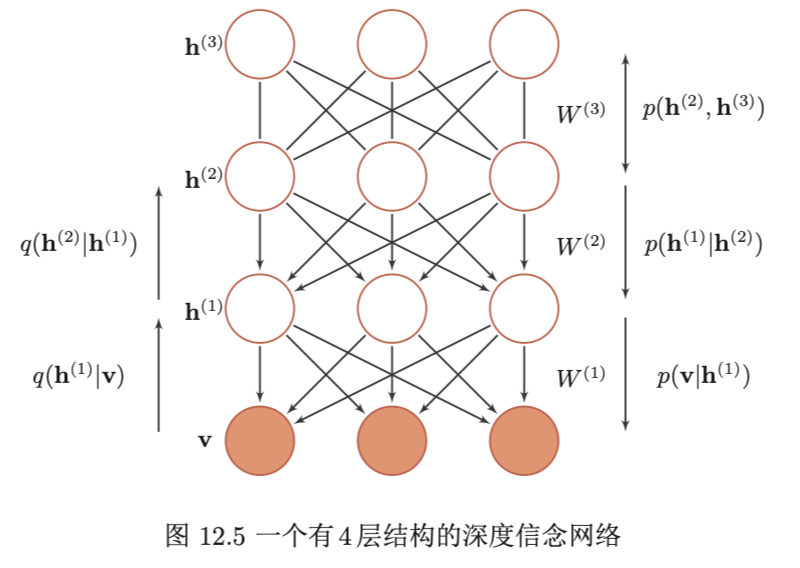p ( h ( l ) ∣ h ( l + 1 ) , . . . , h ( L ) ) = p ( h ( l ) ∣ h ( l + 1 ) ) p(h^{(l)} | h^{(l+1)}, ..., h^{(L)}) = p(h^{(l)} | h^{(l+1)})

p ( v , h ( 1 ) , . . . , h ( L ) ) = p ( v ∣ h ( 1 ) ) ( ∏ l = 1 L − 2 p ( h ( l ) ∣ h ( l + 1 ) ) ) p ( h ( L − 1 ) , h ( L ) ) = ∏ l = 0 L − 1 p ( h ( l ) ∣ h ( l + 1 ) ) p ( h ( L − 1 ) , h ( L ) ) p(v,h^{(1)},...,h^{(L)}) = p(v|h^{(1)})( \prod_{l=1}^{L-2} p(h^{(l)} | h^{(l+1)} ) )p( h^{(L-1)}, h^{(L)}) \\ = \prod_{l=0}^{L-1} p(h^{(l)} | h^{(l+1)} ) p(h^{(L-1)},h^{(L)})

p ( h ( l ) ∣ h ( l + 1 ) ) = σ ( a ( l ) + W ( l + 1 ) h ( l + 1 ) ) p(h^{(l)} | h^{(l+1)}) = \sigma (a^{(l)}+W^{(l+1)} h^{(l+1)})

生成模型

DBN是一个生成模型，可以用来生成符合特定分布的样本。隐变量用来描述在可观测变量之间的高阶相关性。假如训练数据服从分布 p ( v ) p(v) ，通过训练得到一个深度信念网络。

参数学习

DBN最直接的训练方式可以通过最大似然方法使得可观测变量的边际分布 p ( v ) p(v) 在训练集合上的似然达到最大。但在深度信念网络中，隐变量 h h 之间的关系十分复杂，由于“贡献度分配问题”，很难直接学习。即使对于简单的单层Sigmoid信念网络
p ( v = 1 ∣ h ) = σ ( b + w T h ) p(v=1 | h) = \sigma (b + w^Th)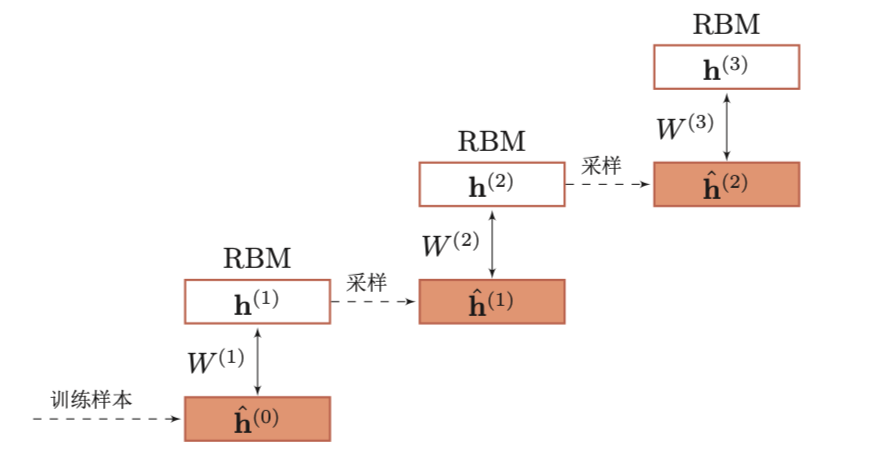p ( h ( i ) ∣ h ( i − 1 ) ) = σ ( b ( i ) + W ( i ) h ( i − 1 ) ) , 1 ≤ i ≤ ( l − 1 ) p(h^{(i)} | h^{(i-1)}) = \sigma( b^{(i)} + W^{(i) h^{(i-1)}} ), 1 \leq i \leq (l-1)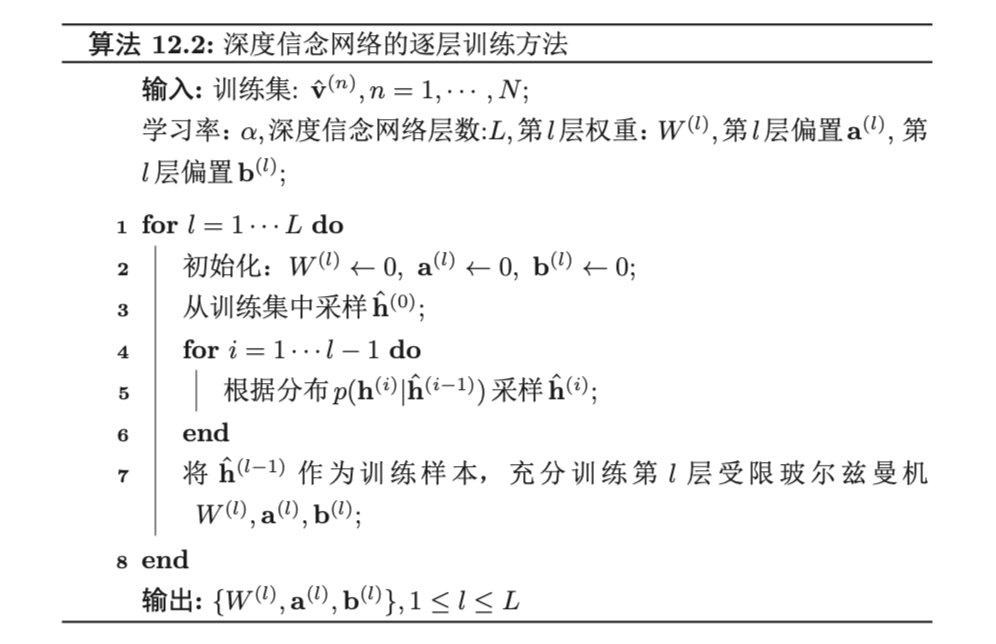• Wake阶段：认知过程，通过外界输入（可观测变量）和向上认知权重，计算每一层隐变量的后验概率并采样。然后，修改下行的生成权重使得下一层的变量的后验概率最大。也就是“如果现实跟我想象的不一样，改变我的权重使得我想象的东西就是这样的”
• Sleep阶段：生成过程，通过顶层的采样和向下的生成权重，逐层计算每一层的后验概率并采样。然后，修改向上的认知权重使得上一层变量的后验概率最大。也就是“如果梦中的景象不是我闹中的相应概念，改变我的认知权重使得这种景象在我看来就是这个概念”
• 交替进行Wake和Sleep过程，直到收敛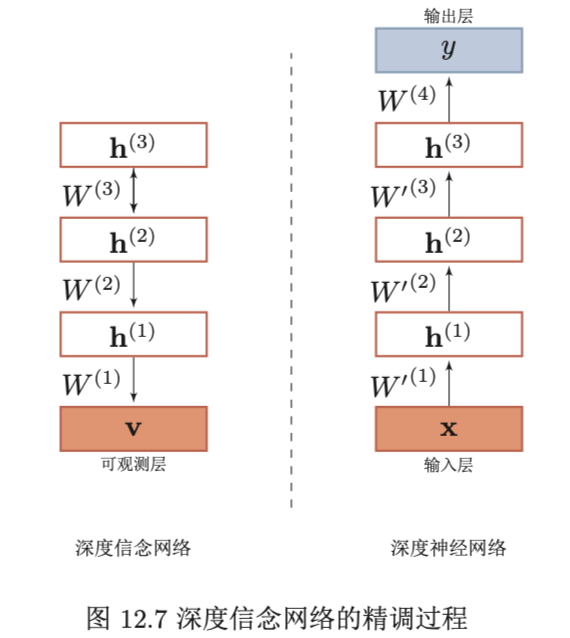总结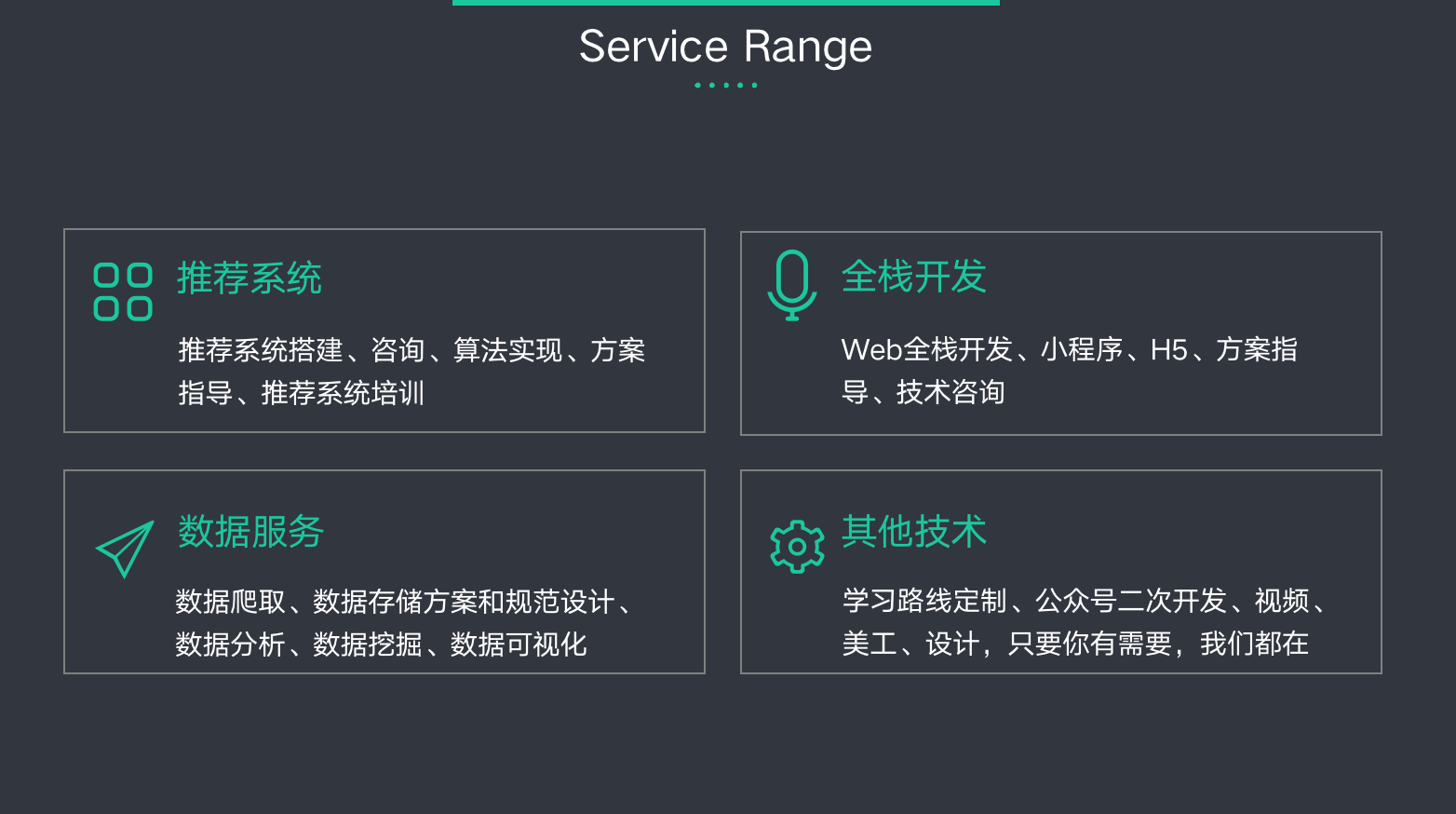12-137万+
03-015902
07-092万+
10-108862
09-19537
08-063万+
11-294218
09-041309
01-17
03-285万+
09-119571
11-253792
08-312万+
08-153189
11-026万+
08-16291
08-051万+¥2 ¥4 ¥6 ¥10 ¥20余额支付 (余额：-- )扫码支付获取中扫码支付点击重新获取扫码支付1.余额是钱包充值的虚拟货币，按照1:1的比例进行支付金额的抵扣。
2.余额无法直接购买下载，可以购买VIP、C币套餐、付费专栏及课程。余额充值